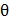# Two masses m andare connected at the two ends of a massless rigid rod of length ll.  The rod is suspended by a thin wire of torsional constant k at the centre of mass of the rod-mass system (sec figure). Because of torsional constant k, the restoring torque is=kfor angular displacement 0.  If the rod is rotated by0 and released, the tension in it when it passes through its mean position will be:a.b.c.d.## Question ID - 50047 :- Two masses m andare connected at the two ends of a massless rigid rod of length ll.  The rod is suspended by a thin wire of torsional constant k at the centre of mass of the rod-mass system (sec figure). Because of torsional constant k, the restoring torque is=kfor angular displacement 0.  If the rod is rotated by0 and released, the tension in it when it passes through its mean position will be:a.b.c.d.3537==Ω=θ0=average velocity

T=mΩ2r1

T=m Ω2=m2=m=I=l2=l2

=r1=Next Question :

A copper wire is stretched to make it 0.5% longer. The percentage change in its electrical resistance if its volume remains unchanged is:

 a. 2.5% b. 0.5% c. 1.0% d. 2.0%